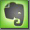Color Depth

Color Bit Depth

One bit can store the information of "on" or "off". With more than one bit a higher graduation, more steps, can be stored. Most digital video formats work with a minimum of 8 bits. This describes bits per color channel, R, G and B. 8 bits used for each color of one pixel adds up to a total of 24 bits (3 x 8). If an alpha channel is used it will need another 8 bits, giving a total of 32 bits for an 8 bit video with alpha channel.
The next higher quality is 10 bits per color channel.
For the sample ratio 4:4:4 the three numbers for the bit depth describe Red, Green and Blue. For instance 8 bit red, 8 bit green, 8 bit blue. For component video (Y' Cb' Cr') with the sample ratios of 4:2:2, 4:2:0, 4:1:1 etc. the bit depth decribes the three component channels, like 8 bit for luminance Y, 8 bits for chrominance Cb' and 8 bits for chrominance Cr'.

Not all possible gradations are used for image information. For 8 bit, of the 256 gradation steps the first 15 are lower than black and the last steps from 236 to 256 are higher than white. The lowest black is step 16 and the highest white is step 135. This leaves enough footroom and headroom. Levels above 235 are also called super-white levels.

 Bits Gradation 1 bit 2 steps 2 bit 4 steps 4 bit 16 steps 8 bit 256 steps 10 bit 1.024 steps 12 bit 4.096 steps 16 bit 65.536 steps 24 bit 16.777.216 steps

A system with 8 bits for each red, green and blue combine for a total of 24 bits for the combined colors. This are 16.777.216 combined gradations for the pixel color.

RGB Color Depth